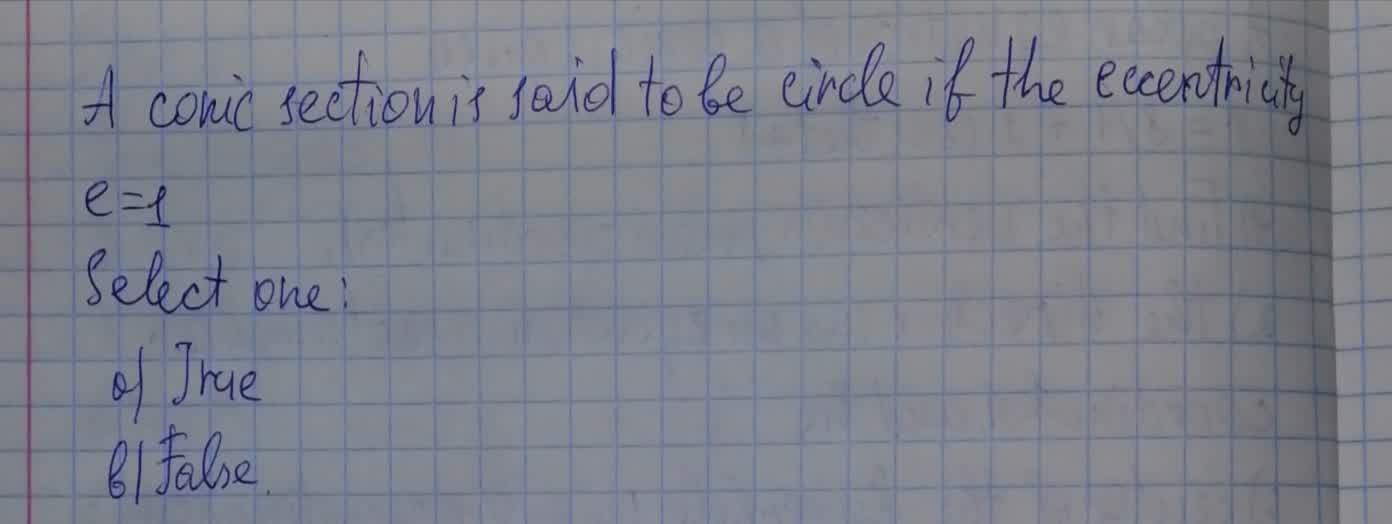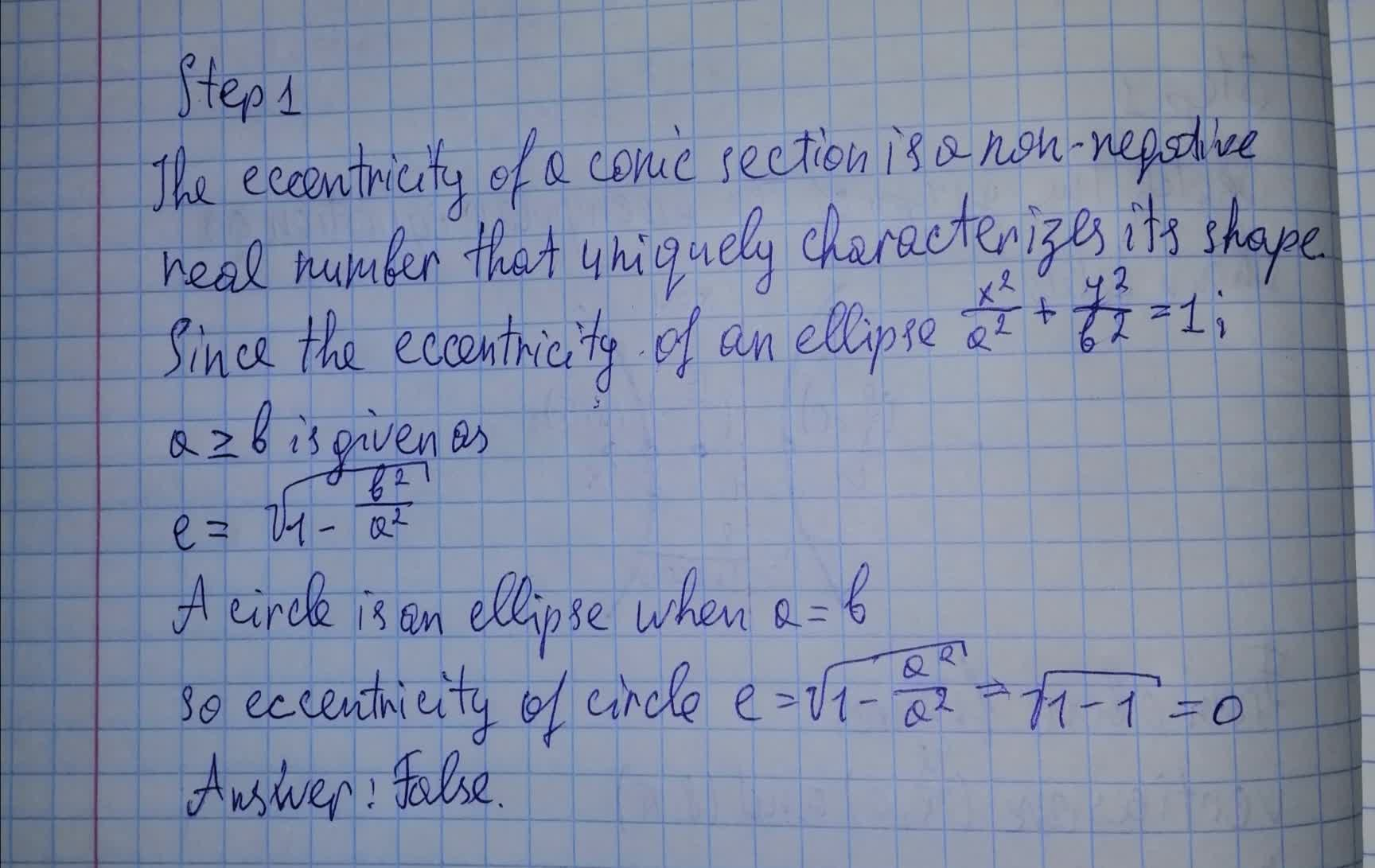A conic section is said to be circle if the eccentricity e=1Rivka Thorpe 2021-08-14 Answered
A conic section is said to be circle if the eccentricity e=1• Questions are typically answered in as fast as 30 minutes

Solve your problem for the price of one coffee

• Math expert for every subject
• Pay only if we can solve itfirmablogF
Explanation of conic section:Not exactly what you’re looking for?Vasquez

Step 1

The eccentricity of a conic section is a non-negative real number that uniquely characterizes its shape

Since the eccentricity of an ellipse $$\frac{x^2}{a^2}+\frac{y^2}{b^2}=1$$;

$$a \geq b$$ is given as

$$e=\sqrt{1-\frac{b^2}{a^2}}$$

A circle is an ellipse when a=b

so eccentricity of circle $$e=\sqrt{1-\frac{a^2}{a^2}}=\sqrt{1-1}=0$$